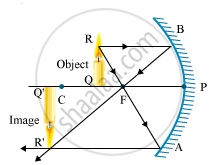# A student wants to project the image of a candle flame on a screen 48 cm in front of a mirror by keeping the flame at a distance of 12 cm from its pole. - Science

A student wants to project the image of a candle flame on a screen 48 cm in front of a mirror by keeping the flame at a distance of 12 cm from its pole.

(a) Suggest the type of mirror he should use.

(b) Find the linear magnification of the image produced.

(c) How far is the image from its object?

(d) Draw ray diagram to show the image formation in this case.

#### Solution

(a) He should use a concave mirror, as it forms a real image on the same side of the mirror.

(b) Object distance, u = -12 cm

Image distance, v = - 48 cm

"Magnification, "m=-v/u=((-48))/((-12))=-4

The minus sign in magnification shows that the image formed is real and inverted.

(c) The image is formed at a distance of 36 cm from the object.

(d)In this case, the image is formed beyond the centre of curvature. This image is real, inverted and enlarged.

Concept: Concave Mirror
Is there an error in this question or solution?
2013-2014 (March) All India Set 2

Share